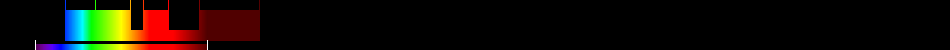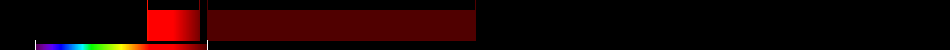# Information for Sensor and Index

## Formula

General Formula $\frac{{\mathrm{NIR}}}{{\mathrm{RED}}+\frac{{b}}{{a}}}$ $\frac{{\mathrm{B4}}}{{\mathrm{B3}}+\frac{{b}}{{a}}}$ Automatic

## Sensor

Name Formosat-2 5 450-900 2-8 0 National Space Organization (NSPO) of the Republic of China (Taiwan) 2004-05-19 yes

## Index

Name Soil-adjusted vegetation index 2 SAVI2 $\frac{{\mathrm{NIR}}}{{\mathrm{RED}}+\frac{{b}}{{a}}}$ The actual gain (a) and intercept (b) values of the soil line rather than assuming them to be 1 and 0, respectively 640:760,780:1400 Original formula

## References